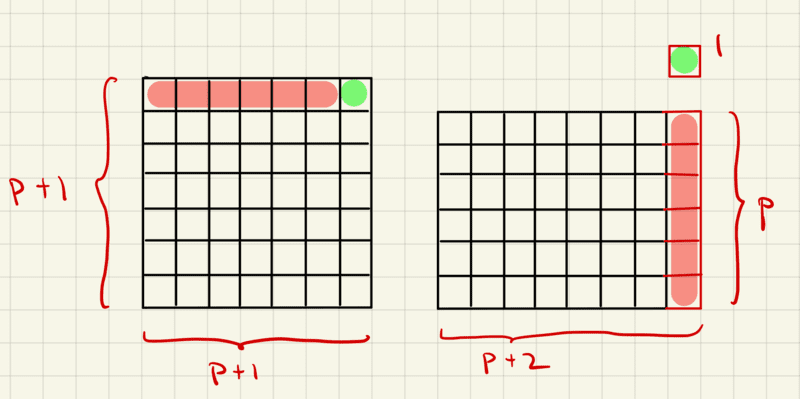# Proof: Twin Primes Always Result in Perfect Squares

• Math100
In summary, if ##p## and ##p+2## are twin primes, adding 1 to their product will always result in a perfect square. This can be seen through the equation ##p(p+2)+1=(p+1)^2##, which is a perfect square. This holds true for any two integers that differ by two, and can be easily visualized geometrically. Therefore, the statement holds regardless of whether the integers are integers or not.f

#### Math100

Homework Statement
If ## 1 ## is added to a product of twin primes, prove that a perfect square is always obtained.
Relevant Equations
None.
Proof:

Suppose ## p ## and ## p+2 ## are twin primes.
Then we have ## p(p+2)+1=p^2+2p+1=(p+1)^2 ##.
Thus, ## (p+1)^2 ## is a perfect square.
Therefore, if ## 1 ## is added to a product of twin primes,
then a perfect square is always obtained.

•fresh_42 and fishturtle1
Looks good to me. Stylistically, one might write "Thus, ##p(p+2)+1## is a perfect square." instead of "Thus, ##(p+1)^2## is a perfect square.".

•Math100
… although the restriction to twin primes is unnecessary. As should be clear from the proof, it holds for any two integers that differ by two.

Edit: There is also a rather intuitive geometric interpretation: Make a square out of (p+1)^2 unit boxes. Take the top row containing p+1 unit boxes and place p of them in a column on the right side of the rectangle, thus leaving you with a rectangle of side lengths p and p+2 with a single leftover unit box.

Edit 2: IllustrationEdit 3: Even easier to see with ##q = p+1##, i.e.,
$$(q-1)(q+1) = q^2 - 1 \quad \Leftrightarrow \quad q^2 = (q-1)(q+1) + 1.$$

Edit 4: The generalisation being cutting a strip of width b from the top of a square of side length a and placing a portion of length a-b of the strip to the right of the square, leaving a rectangle with sides a-b and a+b and a square of side length b:
$$a^2 = (a-b)(a+b) + b^2.$$
Regardless of ##a## and ##b## being integers or not.

Last edited:
•jim mcnamara, SammyS and PeroK
Stylistically, one might write "Thus, ##p(p+2)+1## is a perfect square." instead of "Thus, ##(p+1)^2## is a perfect square.".
This is more than a stylistic point: if you say "Thus, ##(p+1)^2## is a perfect square" then you are saying "because ## p(p+2)+1=p^2+2p+1=(p+1)^2 ## then ##(p+1)^2## is a perfect square" which is not correct (note 1). The words "Then we have" are also not appropriate here because that is saying "because ## p ## and ## p+2 ## are twin primes then ## p(p+2)+1=p^2+2p+1##" which is not correct (note 2).

The proof simply needs to be:
Suppose ## p ## and ## p+2 ## are twin primes.
## p(p+2)+1=p^2+2p+1=(p+1)^2 ##, which is a perfect square.

Note 1: ##(p+1)^2## is a perfect square independent of the fact that ## p(p+2)+1=p^2+2p+1=(p+1)^2 ##.
Note 2: ## p(p+2)+1=p^2+2p+1## independent of whether ## p ## and ## p+2 ## are twin primes.

•fishturtle1 and Mark44